# 2nd PUC Basic Maths Previous Year Question Paper June 2017

Students can Download 2nd PUC Basic Maths Previous Year Question Paper June 2017, Karnataka 2nd PUC Maths Model Question Papers with Answers helps you to revise the complete Karnataka State Board Syllabus and score more marks in your examinations.

## Karnataka 2nd PUC Basic Maths Previous Year Question Paper June 2017

Time: 3.15 hours
Max Marks: 100

Instructions:

• The question paper has 5 parts A, B, C, D and E. Answer all the pats.
• Part-A carries 10 marks), Parts – B carries 20 marks, Part – C carries 3 marks, Part – D carries 30 marks and Part – E carries 1 marks.
• Write the question number properly as indicated in the question paper.

Part – A

Answer all the ten questions: (10 × 1 = 10)

Question 1.
Evaluate $$\left| \begin{matrix} 3200 & 3201 \\ 3202 & 3203 \end{matrix} \right|$$Question 2.
In how many ways can 10 people seated around a table ?
(10 – 1)! = 9!

Question 3.
Write the verbal form of the compound proposition pvq where
P:x is an integer; Q: 5 is odd number.
PVQ = x is an integer or 5 is odd number.

Question 4.
Find the triplicate ration of 3 : 5.
33 : 53 = 27:125Question 5.
A bill was drawn for 3 months was legally due on 18-08-2012. Find the date of drawing of the bill.
Drawn date = L.D.D – B.P – 3 days = 15 – 5 – 12

Question 6.
Find the value of cos3 20° – 3cos 20°.
Cos 3(20) = cos60 = $$\frac { 1 }{ 2 }$$

Question 7.
If the length of the latus rectum of the parabola x2 = 4k y is 8. Find the value of k.
4k = 8 ⇒ k = 2

Question 8.
Evaluate line $$\lim _{x \rightarrow 3}\left(\frac{x^{2}-x}{x-2}\right)$$
$$=\frac{3^{2}-3}{3-2}=\frac{9-3}{1}=6$$

Question 9.
Differentiate : 5ex – loge x – 3√x w.r.t.x.
$$\frac{d y}{d x}=5 e^{x}-\frac{1}{x}-\frac{3}{2 \sqrt{x}}$$

Question 10.
Evaluate ∫sec2 (x – 5)dx.
Tan (x – 5)

Part – B

Answer any ten questions. (10 × 2 = 20)

Question 11.
If A = $$\left[ \begin{matrix} 2 \\ -1 \\ 3 \end{matrix} \right]$$, B = $$\left[ \begin{matrix} 1 & 4 & 2 \end{matrix} \right]$$ find AB and BA.Question 12.
In a party each person shakes hands with everyone else. If there are 25 members in the party, calculate the number the number of hand shakes.
$$25 C_{2}=\frac{25 \times 24}{2 \times 1}=25 \times 12=300$$

Question 13.
A bag contains 6 white beads and 4 red beads. A bead is drawn at random, what is the probability that the bead drawn is white.
P(beaddrawniswhite) = $$\frac{10 C_{1}}{6 C_{1}} \frac{10}{6}=\frac{5}{3}$$

Question 14.
If p,q, r are the propositions with truth values F, T and F repectively. Then find the truth value of the ; compound proposition p → (q → r).
P → (q → r)
F → (T → F)
F → F = T

Question 15.
If a : b = 4 : 5. Find $$\frac{3 a+2 b}{3 a-2 b}$$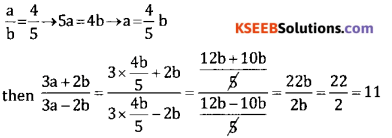Question 16.
Banker’s gain on a bill due after 6 months at 4% p.a. is 24. Find true discount and Banker’s discount,
Given B.G = 24. T = 6 months, = $$\frac { 1 }{ 2 }$$ yr, r = 4% = 0.0
BG = TD + r
24 = TD × $$\frac { 1 }{ 2 }$$ × 0.04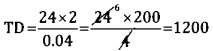TD = 1200
BG = BD – TD ⇒ BD = BG + TD = 24 + 1200 = 1224

Question 17.
Prove that $$\frac{\sin 3 A}{1+2 \cos 2 A}$$ = sin A.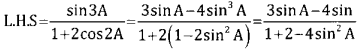= Sin A = R.H.S

Question 18.
If tan A = $$\frac { 5 }{ 6 }$$ tan B = $$\frac { 1 }{ 11 }$$ Show that A + B = $$\frac{\pi}{4}$$
Consider tan (A+B) = $$\frac{\tan A+\tan B}{1+\tan A \cdot \tan B}$$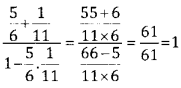Tan (A + B) = 1 ⇒ A + B = $$\frac{\pi}{4}$$Question 19.
Find the equation of the circle whose centre is at (4,-2) and passing through the origin (0,0)
C = (4,-2) & r = $$\sqrt{4^{2}+(-2)^{2}}=\sqrt{16+4}=\sqrt{20}$$
Equation of the circle (x + 4)2 + (y + 2)2 = (√ 20)
⇒ x2 + 16 – 8x + y2 + y + 4y – 20 = 0
⇒ x2 + y2 – 8x + 4y = 0

Question 20.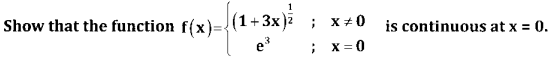$$\lim _{x \rightarrow 0}$$ f(x) = $$\lim _{x \rightarrow 0}$$ (1 + 3x)1/3 = e3 = f(0)
∴ f(x) is continuous at x = 0

Question 21.
If siny = x sin(a+y), prove that $$\frac{d y}{d x}=\frac{\sin ^{2}(a+y)}{\sin a}$$Question 22.
If s = at3 + bt, find a and b given that when t = 3, velocity is 0 and the acceleration is 14 units.
If s = at3 + bt velocity = $$\frac{\mathrm{ds}}{\mathrm{dt}}$$ = 3at2 + b = 0
Acceleration = $$\frac{\mathrm{dv}}{\mathrm{dt}}$$ = 6at = 14
At t = 3sec, 6a × 3 = 14 ⇒ a = $$\frac{14}{18}=\frac{7}{9}$$Question 23.
Evaluate $$\int \frac{3^{x} \log 3}{\left(3^{x}+1\right)} d x$$
= log(3x + 1) + C ∴ $$\int \frac{f^{1}(x)}{f(x)} d x$$ = log(3x +1)

Question 24.
Evaluate $$\int_{1}^{2} \frac{1}{(2 x+3)} d x$$Part – C

Answer any ten questions: (10 × 3 = 30)

Question 25.
If 2A + B = $$\left[ \begin{matrix} 3 & -1 \\ -2 & 5 \end{matrix} \right]$$ and A – 2B = $$\left[ \begin{matrix} 4 & 2 \\ -1 & 5 \end{matrix} \right]$$ then find A and B.

Question 26.
Solve $$\left| \begin{matrix} 2+x & 3 & -4 \\ 2 & 3+x & -4 \\ 2 & 3 & -4+x \end{matrix} \right| =0$$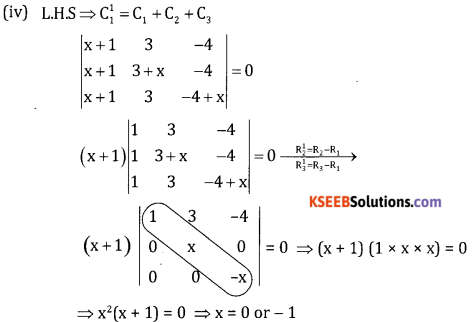Question 27.
Find the number of permutations of the letters of the word ‘ENGINEERING’ How many of these
i. Begin with E and end with E
ii. Have all the 3E’s together?
Total-11, N – 3, G – 2, 1 – 2, E = 3
Total permutations $$\frac{11 !}{3 ! \times 3 ! \times 2 ! \times 2 !}$$=
I. Number of permutations which begin with E & end with E is = $$\frac{9 !}{3 ! \times 2 ! \times 2 !}$$
II. Number of Permutations in Which all 3E’s are together (Total 11 – 3 + 1 = 9′) = $$\frac{9 !}{3 ! \times 2 ! \times 2 !}$$

Question 28.
A die is thrown, If E is the event “the number appearing is a multiple of 3” and F be the event “the number appearing is even”. Then find whether E are Fare independent.
S = {1,2,3,4,5,6}
E = {3,6), F = {2,4,6}
N(S) = 6, n(E) = 2 n(F) = 3
P(E) = $$\frac{2}{5}=\frac{1}{3}$$ P(E) = $$\frac{3}{6}=\frac{1}{3}$$
They are not independent

Question 29.
Two tap can separately fill a tank in 12 minutes and 15 minutes respectively. The tank when full can be emptied by a drain pipe in 20 minutes. When the tank was empty all the three were opened simultaneously in what time will the tank be filled up?
A fills tank in 12 mins → In 1 min A will fill 1/12 of tank.
B fills tank in 15 mins → In 1 min B will fill 1/15 of tank.
Lets say C can alone empty the tank in x mins → In 1 min C will empty 1/x of tank.
According to question, if all taps are opened tank will fill in 20 mins.
In each min 1/20 tank is filled which is due to A, B and C. Now A and B fill the tank will C empty the tank.

1/12 + 1/15 – 1/x = 1/20

1/12 + 1/15 – 1/20 = 1/x

(5 + 4 – 3)/60 = 1/x

6/60 = 1/x

x = 10

Question 30.
Bharath bought a shirt for ₹ 336 including 12% sales tax and a neck tie for 110 including 10% sales tax. Find the printed price of shirt and neck tie together.
Given S.P = ₹ 336, S.P (neck tie) = 110/
Let C.P of shirt = x, & C.P of neck tie = y
Here, setting price of shirt = C.P + 12% of C.P
336 = x + $$\frac{12 x}{100}$$
336 = $$=\frac{112 x}{100}$$ ⇒ x = $$\frac{33600}{112}$$
Selling price of neck tie = C.P + 10% of C.P
110 = y + $$\frac{10 y}{100}$$
110 = $$\frac{10 y}{100}$$ ⇒ $$\frac{1100}{11}$$ = 100
∴ Printed price of shirt & neck tie = 300 + 100 = ₹ 400

Question 31.
A Banker pays ₹ 4,250 on a bill of ₹ 5,000. 146 days before the legally due date. Find the rate of discount charged by the Banker.
Given F = ₹ 5000, Discounted Value = ₹ 4,520
t = 146 days = $$\frac{146}{365}$$ year,r = ?
BD = F – Discounted value = 5000 – 4520 = ₹ 480
BD = F/r ⇒ 480 = $$\frac{5000 \times 146 \times r}{365}$$
∴ r = $$\frac{480 \times 365}{5000 \times 146}$$ = 0.24 × 100=24

Question 32.
Rakshith decides to invest in TCS shares which are selling at 2020 per share. How many money is required to purchase 10 shares if the brokerage is 0.5%?
Selling price of 10 shares at 2020 per share = 20,200
0.5 Brokerage = $$\frac{0.5}{100}$$ × 20,200 = ₹ 101
100 Amount required to purchase = 20,200 + 101 = 20,301

Question 33.
Find the equation of the parabola if its focus is (4,0) and directrix is x = -4. Also find the equation of the tangent at the vertex.
Given Directrix As x = -4 & Focus = (4,0) ⇒ a = 4
The standard equation is y2 = 4ax ⇒ y2 = 4 × 4 x X = 16x
∴ Equation of the parabola = 16xQuestion 34.
If x = a cos4θ, y = a sin46 show that $$\frac{d y}{d x}-\tan ^{2} \theta$$
x = a cost4θ & y = a sin4
Differentiate both w.r.t θ we get
$$\frac{d y}{d \theta}$$ = a.4cos3θ.sin θ, $$\frac{d y}{d \theta}$$ = 2.4 sin3θ. cosθQuestion 35.
The side of an equilateral triangle is increasing at the rate of √3 cm/s. Find the rate at which its area is including when its side is 200 cms.
Given $$\frac{\mathrm{dx}}{\mathrm{dt}}$$ = $$\sqrt{3}$$ cm/sec., x = 2 meters, $$\frac{\mathrm{dA}}{\mathrm{dt}}$$ = ?
Area of equilateral Δle = A = $$\frac{\sqrt{3}}{4}$$ x2
$$\frac{d A}{d t}=\frac{\sqrt{3}}{4} \cdot 2 x \frac{d x}{d t}$$
= $$\frac{\sqrt{3}}{4}$$ . 2. 200. $$\sqrt{3}$$ = 300cm2 / sec.

Question 36.
Find two positive numbers whose sum is 14 and the sum of whose square is minimum.
Let the two numbers be x and y
Given x + y = 14 & S = x2 + y2 where y = 14 – x
∴ S = x2 + (14 – x)2 = x2 + 142 + x2 – 28x = 2x2 – 28x + 142
$$\frac{d s}{d x}$$ = 4x – 28 → (1) $$\frac{d s}{d x}$$ = 0 ⇒ 4x – 28 = 0 ⇒ x = 7
$$\frac{\mathrm{d}^{2} \mathrm{s}}{\mathrm{d} \mathrm{x}^{2}}$$ = 4 > 0, sum is minimum.
∴ y = 14 – x = 14 – 7 = 7
∴ the two positive number are 7 & 7.

Question 37.
Evaluate $$\int \frac{1+\cos 2 x}{1-\cos 2 x} d x$$
= $$=\int \frac{2 \cos ^{2} x}{2 \cos } d x$$ = ∫cos2 dx = ∫(cosec2x – 1)dx = – cos x – x + c

Question 38.
Evaluate $$\int_{0}^{1}(6 x+1) \sqrt{3 x^{2}+x+5 d x}$$
Put 3x2 + x + 5 = t.
(6x + 1) dx = dt
When x = 0 then t = 5
When x = 1 then t = 9
$$\left.\int_{0}^{1}(6 x+1)(\sqrt{3 x^{2}+x+5}) d x=\int_{0}^{1} \sqrt{t} d t=\frac{2}{3} t^{\frac{3}{2}}\right]_{5}^{7}$$
$$=\frac{2}{3}\left(9^{\frac{3}{2}}-5^{\frac{3}{2}}\right)=\frac{2}{3}(27-5 \sqrt{5})$$Part-D

Answer any six questions: (6 × 5 = 30)

Question 39.
Simplify (√2 + 1)6 – (√2 – 1)6 using Binomial theorem.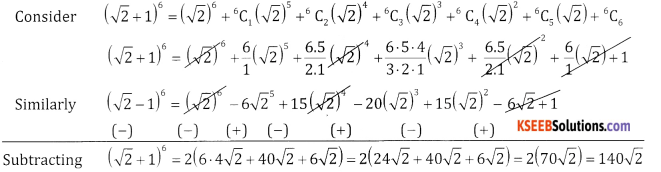Question 40.
Resolve $$\frac{2 x^{2}-4 x+1}{(x-2)(x-3)^{2}}$$ into partial fractions.
2x2 – 4x + 1 = A[x – 3)2 + B(x – 2) (x-3) + C (x – 2)
Put x = 2, 8-8 + 1 = A(-1)2 = A = 1
Put x = 3, 18 – 12 + 1 = A(0) + B(0) + C(3 – 2) = 7 = C
Comparing coefficient of x2 = 2 = A + B = B = 2-A = 2 -1 =1
∴ $$\frac{2 x^{2}-4 x+1}{(x-2)(x-3)^{2}}=\frac{1}{x-2}+\frac{1}{x-3}+\frac{7}{(x-3)^{2}}$$

Question 41.
Prove that (p ∨q)∧(~P∧q~) is a contradiction.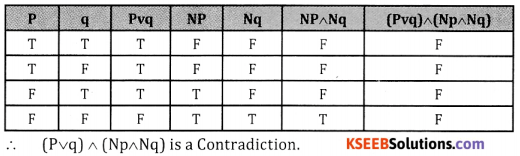Question 42.
Divide ₹ 1,647 into three parts such that $$\frac { 3 }{ 7 }$$ th of the first, $$\frac { 2 }{ 3 }$$ rd of second and $$\frac { 4 }{ 5 }$$ th of the third are equal..
Let the 3 parts be A,B & C
Given $$\frac{3}{7} A=\frac{2}{3} B \Rightarrow \frac{A}{B}=\frac{2}{7} \times \frac{7}{3}=\frac{14}{9}$$
∴ A : B = 14 : 9
Also given $$\frac{2}{3} B=\frac{4}{5} \Rightarrow \frac{B}{C}=\frac{4}{5} \times \frac{3}{2}=\frac{12}{10}=\frac{6}{5}$$
∴ B : C = 6 : 5
Multiple (1) by 2 and (2) by 3 we get A : B = 28 : 18 and B : C = 18 : 5
∴ A : B : C = 28 : 18 : 15
∴ 28x + 18x + 15x = 1647
⇒ 61x = 1647 ⇒ x = $$\frac{1647}{61}$$ = 27
∴ A receives = 28 × 27 = ₹ 3 756
B receives = 18 × 27 = ₹ 486
Creceives = 15 × 27 = ₹ 405Question 43.
A company requires 100 hours to produce the first 10 units at 15/hour. The learning effect is 80%. Find the total labour cost to produce a total of 160 units.
Given
a = 100 hrs. 10 units = 1 lot
∴ 160 units = 16 lotsy = axb
log y = log a + b log x
= log 100 – 0.3219 log 16
= 2 – 0.3219 1.2041 = 2 – 0.3875 = 1.625
y = A(1.6125) = 40.98 hours/unit
∴ Total time required for 160 units or 16 lots = 40.98 16 = 655.68 hrs.
∴ Total cost to produce 16 units at ₹ 15/hr. = 655.68 × 15 = ₹ 9835.20
Table methodTotal time for 16 lots = 655.36 hrs.
∴ Total cost to produce 16 lots at ₹ 15/hr. = 655.36 × 15 = ₹ 19830.4.

Question 44.
Solve the following L.P.P. graphically maximize z = 60x + 15y subject to the constraints x + y ≤ 50, 3x + y ≤ 90, x ≥ 0, y ≥ 0.
Consider line x + y = 50
Put x = 0, y = 50, (0,50)
Put y = 0, x = 50 (50,0)
Consider the line 3x + y =- 90
Put x = 0, y = 90 (0,90)
Put y = 0, x = 30 (30,0)
Plot the above lines on the graph we get OABC is the solution region≥ is maximum at c (30,0)
zmax = 1800Question 45.
Prove that sin 20° sin 40° sin 60° sin 80°= $$\frac { 3 }{ 16 }$$
L.H.S=sin 60° (sin 40° sin 20° )sin 80°Question 46.
Find the equation of the circle passing through the points (5,1) and (3,4) and has its centre on the x – axis.
Let the required equation of the circle is x2 + y2 + 2gx + 2fy + c = 0
It passes through (5, 1) & (3, 4)
(5, 1) (5)2 + (1)2 + 2g(5) + 2f (1) +C = 0
10g + 2f + C + 26 = 0 …..(1)
(3,4) 32 + 42 + 2g (3) + 2f (4) +c= 0
68 + 8f + C + 25 = 0 …..(2)
& the centre (- g, – f) lies on x-axis ⇒ f = 0 ….(3)
Solving 1 & 2 we get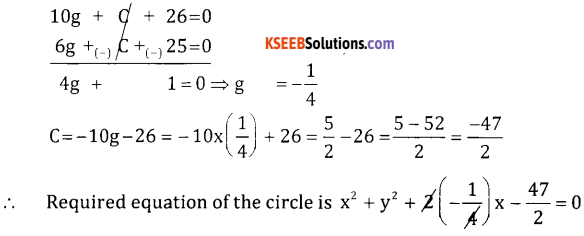⇒ 2x2 + 2y2 – x – 47 = 0.

Question 47.
If y = $$(x+\sqrt{x^{2}+1})$$ show that(x2 – 1}y2 + xy1 – y = 0.
y = x + $$\sqrt{x^{2} – 1}$$ differentiate w.r.t x∴ (x2 – 1) y12 = y2 Differentiate agin w.r.t x
(x2 – 1)2y1y2 + y12 (2x) = 2y .y1 (÷ 2y1 we get)
(x2 – 1)y2 + xy1 – y = 0 Hence proved

Question 48.
Find the area of the region between the parabolas y2 = 4x and x2 = 4y.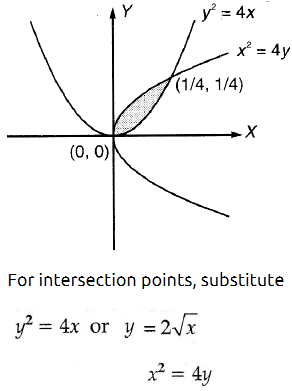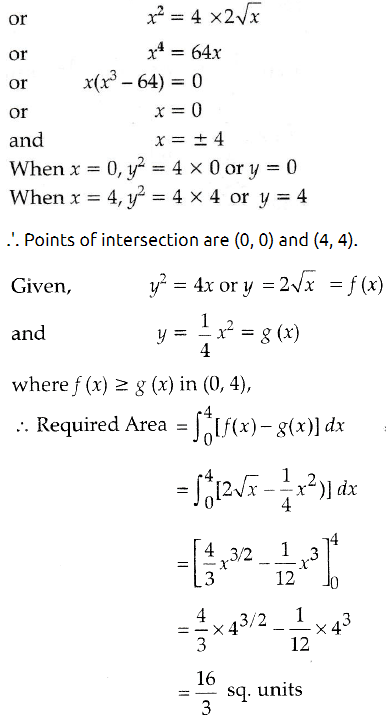Part – E

Answer any one question: (1 × 10 = 10)

Question 49.
(a) A school wants to award its students for the values of punctuality, good behavior and hardwork with a total cash award ₹ 6,000. Three times the award money for hardwork added to that given for punctuality amounts to ₹ 11,000. The award money for punctuality and hardwork together is double the one given for good behavior.

Represent the above situation algebraically and also find the award money for each value using matrix method.

(b) The total revenue function is given by R = 400x – 2x2 and the total cost function given by C = 2x2 + 40x + 4000 find
i. The marginal revenue and marginal cost function.
ii. The average revenue and average cost.
(a) Let the values of punctuality, Good Behaviour and Hard work be denoted by x,y & z receptively
Given x + y +z = 6000 ———1
X + 3z = 11000 ———-2
X + z = 2y ⇒ x – 2y + z = 0 ——- 3
Solve these equations by matrix method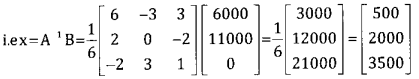Thus x = ₹ 500, y = ₹ 2000 & z = ₹ 3500

(b) (i) MR = $$\frac{d}{d x}$$ (TR) = $$\frac{d}{d x}$$ (400x – 2x2) = 400 – 4x
MC = $$\frac{d}{d x}$$ (TC) = $$\frac{d}{d x}$$(2x2 + 40x + 400 )= 4x – 40

(ii) $$\mathrm{AR}=\frac{\mathrm{TR}}{\mathrm{x}}=\frac{400 \mathrm{x}-2 \mathrm{x}^{2}}{\mathrm{x}}=400-2 \mathrm{x}$$
$$A C=\frac{T R}{x}=\frac{2 x^{2}+40 x+4000}{x}=2 x+40+4000$$

Question 50.
(a) Prove that if $$\lim _{x \rightarrow a}\left(\frac{x^{n}-a^{n}}{x-a}\right)$$ = nan-1 for all values of’n’.

Case 1: Let n be a positive integer.
xn – an = (x-a)(xn-1 + xn-2. a + xn-3. a2 + ———- + 2n-1)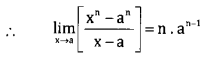Case 2: Let n be a negative integer
put n = -m, m > 0Case 3: Let n = p/q where p and q are integers and q ≠ 0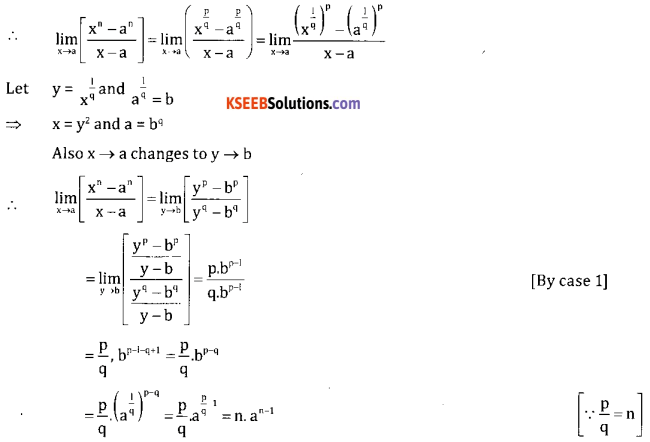Hence it proved for al the values of n

(b) A person is at the top of a tower 75 feet high. From there he observes a vertical pole and finds the angles of depressions of top and bottom of the pole which are 30° and 60° respectively. Find the height of the pole.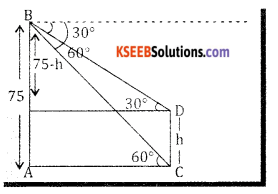width=”278″ height=”194″ />
Let AB = Tower, CD = Pole = h
From triangle ABC we get
tan 60° = $$\frac{A B}{A C} \Rightarrow \frac{75}{A C}=\sqrt{3}$$
⇒ AC = $$\frac{75}{\sqrt{3}} ….. (1)$$ …. (1)

From triangle BDE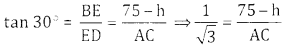⇒ AC = $$\sqrt{3}(75-h)$$

From 1 and 2 we get
$$\frac{75}{\sqrt{3}}=\sqrt{3}(75-h) \Rightarrow 75=(\sqrt{3})^{2}(75-h)$$
75 = 225 – 3h 3h ⇒ 150 ⇒ h = 50
∴ Height of the pole -50ft

error: Content is protected !!